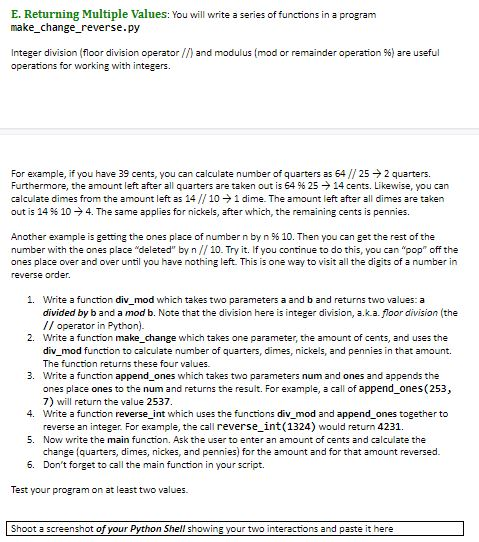# E. Returning Multiple Values: You will write a series of functions in a program make_change_reverse.py Integer division (floor division operator//) and modulus (mod or remainder operation 96 ) are useful operations for working with integers For example, if you have 39 cents, you can calculate number of quarters as 64//25 2 quarters. Furthermore, the amount left after all quarters are taken out is 64 % 25 14 cents . Likewise, you can calculate dimes from the amount left as 14//101 dime. The amount left after all dimes are taken out is 14 % 104. The same applies for nickels, after which, the remaining cents is pennies. Another example is getting the ones place of number n by n 9% 10. Then you can get the rest of the number with the ones place "deleted" by n//10. Try it. If you continue to do this, you can "pop" off the ones place over and over until you have nothing left. This is one way to visit all the digits of a number in reverse order 1. Write a function div_mod which takes two parameters a and b and returns two values: a divided by b and a mod b. Note that the division here is integer division, a.ka. floor division (the operator in Python). Write a function make_change which takes one parameter, the amount of cents, and uses the div_mod function to calculate number of quarters, dimes, nickels, and pennies in that amount. 2. The function returns these four values. Write a function append ones which takes two parameters num and ones and appends the ones place ones to the num and returns the result. For example, a call of append_ones (253, 7) will return the value 2537 Write a function reverse int which uses the functions div_mod and append_ones together to reverse an integer. For example, the call reverse int(1324) would return 4231 3. 4. 5. Now write the main function. Ask the user to enter an amount of cents and calculate the change (quarters, dimes, nickes, and pennies) for the amount and for that amount reversed 6. Don't forget to call the main function in your script. Test your program on at least two values. Shoot a screenshot of your Python Shell showing your two interactions and paste it here

Question

How do repeat the cents value? For example if I use the argument cents(1234), it should print 4321 (backwards as you can see) but I can't see to do that with my code. Thankshelp_outlineImage TranscriptioncloseE. Returning Multiple Values: You will write a series of functions in a program make_change_reverse.py Integer division (floor division operator//) and modulus (mod or remainder operation 96 ) are useful operations for working with integers For example, if you have 39 cents, you can calculate number of quarters as 64//25 2 quarters. Furthermore, the amount left after all quarters are taken out is 64 % 25 14 cents . Likewise, you can calculate dimes from the amount left as 14//101 dime. The amount left after all dimes are taken out is 14 % 104. The same applies for nickels, after which, the remaining cents is pennies. Another example is getting the ones place of number n by n 9% 10. Then you can get the rest of the number with the ones place "deleted" by n//10. Try it. If you continue to do this, you can "pop" off the ones place over and over until you have nothing left. This is one way to visit all the digits of a number in reverse order 1. Write a function div_mod which takes two parameters a and b and returns two values: a divided by b and a mod b. Note that the division here is integer division, a.ka. floor division (the operator in Python). Write a function make_change which takes one parameter, the amount of cents, and uses the div_mod function to calculate number of quarters, dimes, nickels, and pennies in that amount. 2. The function returns these four values. Write a function append ones which takes two parameters num and ones and appends the ones place ones to the num and returns the result. For example, a call of append_ones (253, 7) will return the value 2537 Write a function reverse int which uses the functions div_mod and append_ones together to reverse an integer. For example, the call reverse int(1324) would return 4231 3. 4. 5. Now write the main function. Ask the user to enter an amount of cents and calculate the change (quarters, dimes, nickes, and pennies) for the amount and for that amount reversed 6. Don't forget to call the main function in your script. Test your program on at least two values. Shoot a screenshot of your Python Shell showing your two interactions and paste it here fullscreen

### Want to see this answer and more?

Step-by-step answers are written by subject experts who are available 24/7. Questions are typically answered in as fast as 30 minutes.*